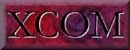### 1. Introduction

Data on the scattering and absorption of photons (x-rays, gamma rays, bremsstrahlung) are required for many scientific, engineering and medical applications. The number of materials for which photon cross sections are needed is large and ever increasing. Available tables [1-11] usually include cross sections for many (but not all) elements. Some tables [1,2,6,11] also contain data for a limited number of compounds and mixtures. In practice it is not possible to meet all cross-section requirements adequately by means of printed tables. Moreover, the cross sections are often needed at photon energies other than those included in the tables.

Photon cross sections for compounds can of course be obtained rather accurately (except at energies close to absorption edges) as weighted sums of the cross sections for the atomic constituents. However, the required numerical work is tedious, and the task is further complicated by the fact that photoabsorption cross sections and total attenuation coefficients are discontinuous at absorption edges. The presence of these discontinuities makes it desirable that cross section tables for compounds include photon energies immediately above and below all the absorption edges for all the atomic constituents, and this requires much additional interpolation.

A convenient alternative approach is to generate the cross sections and attenuation coefficients for compounds and mixtures as needed. This paper describes a web program called XCOM which carries out this task quickly for any element, compound or mixture, at energies between 1 keV and 100 GeV.

The XCOM program can generate cross sections on a standard energy grid (spaced approximately logarithmically), or on a grid selected by the user, or for a mix of both grids. Cross sections at energies immediately above and below all absorption edges are automatically included. XCOM provides two forms of output: (a) tables which correspond closely in format to existing tables in the literature; (b) graphical display of the tabular data.

The program provides total cross sections and attenuation coefficients as well as partial cross sections for the following processes: incoherent scattering, coherent scattering, photoelectric absorption, and pair production in the field of the atomic nucleus and in the field of the atomic electrons. For compounds, the quantities tabulated are partial and total mass interaction coefficients, which are equal to the product of the corresponding cross sections times the number of target molecules per unit mass of the material. The reciprocals of these interaction coefficients are the mean free paths between scatterings, between photo-electric absorption events, or between pair production events. The sum of the interaction coefficients for the individual processes is equal to the total attenuation coefficient. Total attenuation coefficients without the contribution from coherent scattering are also given, because they are often used in gamma-ray transport calculations.

The interaction coefficients and total attenuation coefficients for compounds or mixtures are obtained as sums of the corresponding quantities for the atomic constituents. The weighting factors, that is, the fractions by weight of the constituents, are calculated by XCOM from the chemical formula entered by the user. For mixtures, however, the user must supply the fractions by weight of the various components.

Some limitations should be noted. The cross sections for elements in the XCOM database pertain to isolated neutral atoms, and do not take into account molecular and solid-state effects which modify the cross sections, especially in the vicinity of absorption edges. Relatively small cross sections, such as those for Delbruck scattering, two-photon Compton scattering or photo-meson production, are not included. Also omitted is the nuclear photoeffect which, in the giant-dipole resonance region from 5 MeV to 30 MeV, can contribute a few percent to the total attenuation coefficient. Finally, XCOM does not calculate energy absorption coefficients that represent the conversion of photon energy to kinetic energy of secondary Compton-, photo-, and pair-electrons.

Introduction   |   Database for Elements   |   Interpolation and Combination   |   Using the Program   |   References